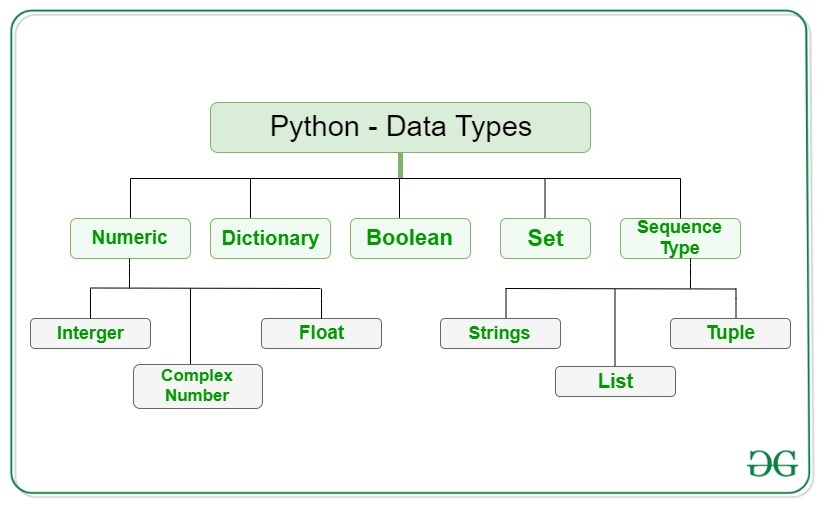### What is a Python List?

Python lists are built-in data types that are used to store multiple items in a single variable.

### Creating a Python List

The first step involves selecting the name or variable which is then equated to square brackets.  Before we add anything between the brackets this is known as a blank list and it could be useful depending on what you’re doing with your code.In this example, we are going to create a list called “bears” and we will populate the list manually with string or text. However, a list can be of anything like numbers, text, dictionaries or even other Python lists.Credit: GeeksforGeeks

The list will contain the following [‘Polar’, ‘Brown’, ‘Panda’, ‘Grizzly’], we have now constructed a list and we can proceed to print out the list as shown below.

And then inside the parentheses, we need to type the element that we want to add to the list, in this case, ‘Koala’. This is going to add the element to the end of the list. If we now print out the list ‘bears’ again we’ll see it’s added to the end of the list. Adding elements to a Python list

Now to do that we use the append function. So to add an item to our Python list, we simply type the name of the list that we want to add something to and we do dot append. This is going to add something to the end of our list. Now it is important to know that it goes to the end of the list because the list is actually an ordered data type.

Note that If we put the print statement before we do the append function we will obtain the first list that does not contain ‘Koala’. As shown in the code below.

### Removing Item(s) from a Python List

Now to remove an item from a list we simply want to use pop. Now pop does what it actually sounds like which is popping the item out of the list. We also create a variable to save the popped item.This can be quite useful and is definitely worth knowing. Let’s proceed to create a variable that goes by the name “item_popped” and then equate it to “bears.pop” with parentheses at the end. Now to remove an item from the list all that we ought to do is give the index number of the item in the list that we want to remove.

Remember that python is a Zero index language therefore it means that items in the Python list start from index zero.

Note that when we print the list out again afterwards we can see that the item ‘Polar’ will now have been removed but we still have it stored in the variable ‘item_popped’.

### Slicing and Indexing a Python List

Now to index an item in a list we will say the item is equal to bears and we’re going to do the square brackets to access each part of it with our index. As we just mentioned python is a zero index language. So if I do zero and then I print out the item in index zero, this is going to now be the first item in this list.

Now you can actually use negative numbers as well. For instance, a minus one is an item at the end of the list. So the first one is a zero and then minus one will go to the actual end.

This is really handy if you wanted to access an item at the end of the list without working out how long it is and then doing that number, this will give us Koala as demonstrated in the code below.

To access multiple parts of the data we can use a slicer. This is going to separate two numbers with a colon and it’s going to get the information in between those two indexes.

For example, if we place [0:2] we are going to get the first and second items in the list because it is up to but not including 2. The output of this code is a new list with those two items in it.Now we can actually just remove the zero because then the code will then just interpret that as the beginning of the Python list. So we’ll get the same results. Furthermore, we can actually do this the other way around as well and we can say it will have everything from the second, all the way to the end of the list. So that’s going to give us the last three items from the list as the output.

In our Programming Tutorials series, you’ll find useful materials which will help you improve your programming skills and speed up the learning process.

Would you like to learn how to code, online? Come and try our first 25 lessons for free at the CodeBerry Programming School.